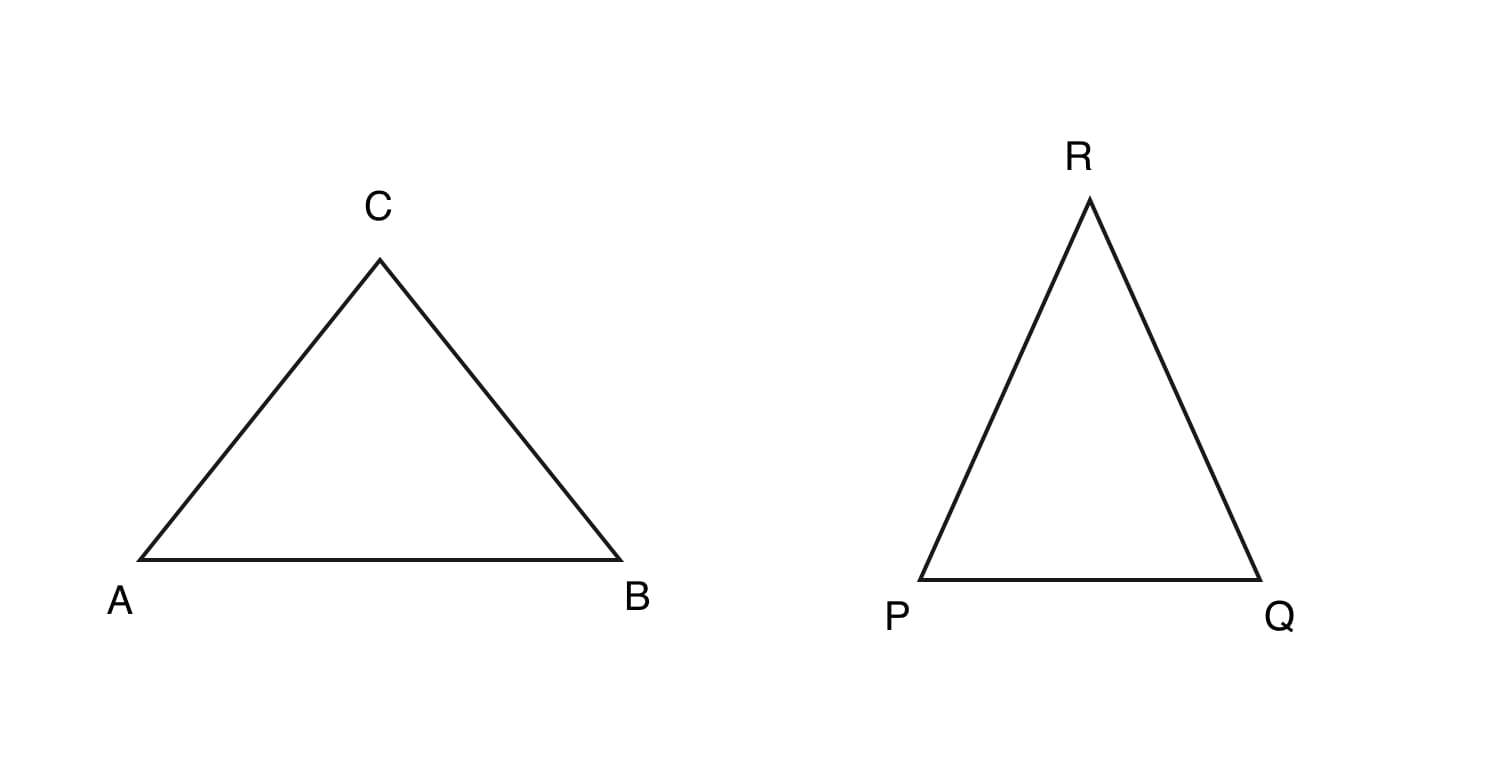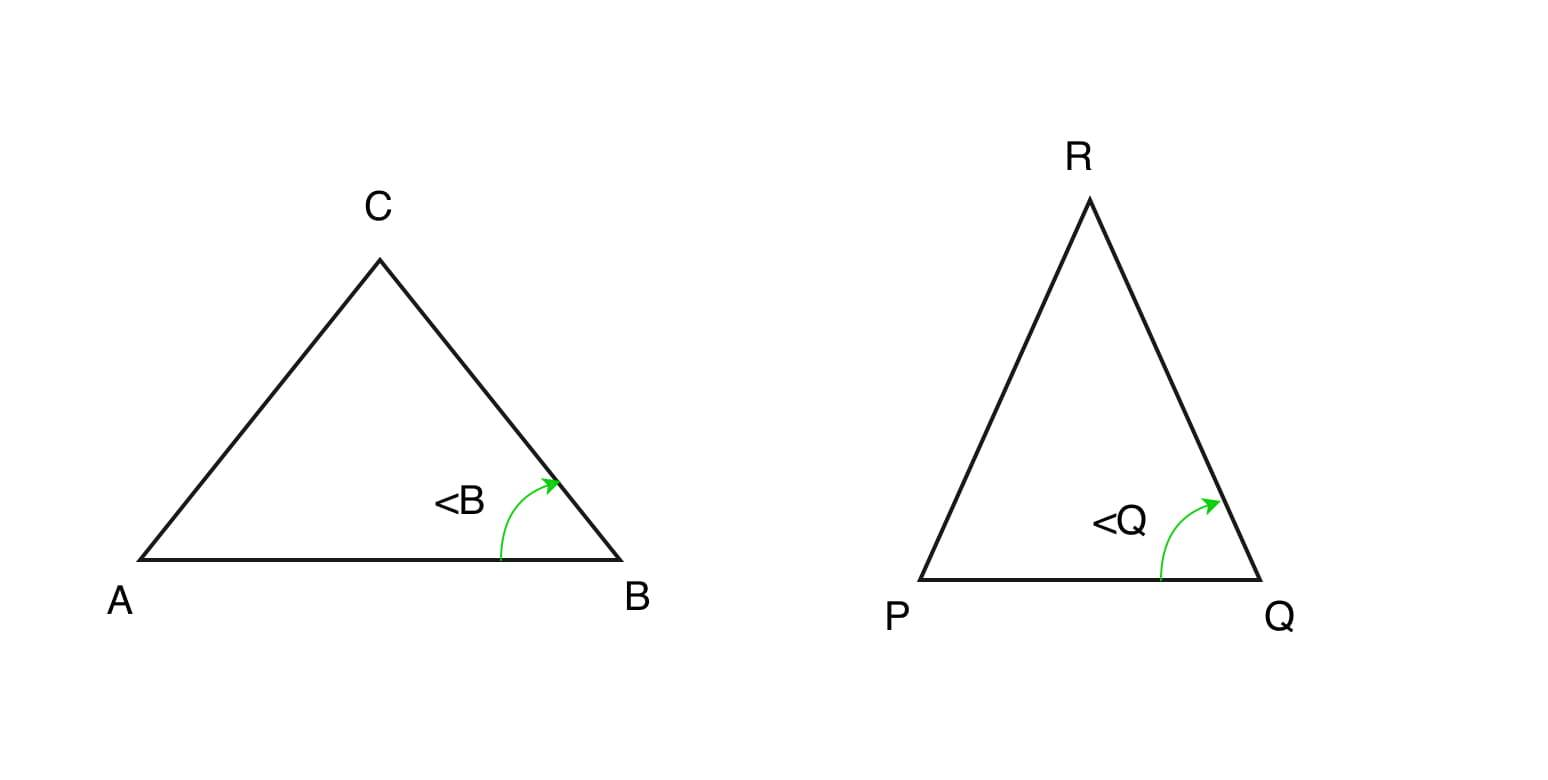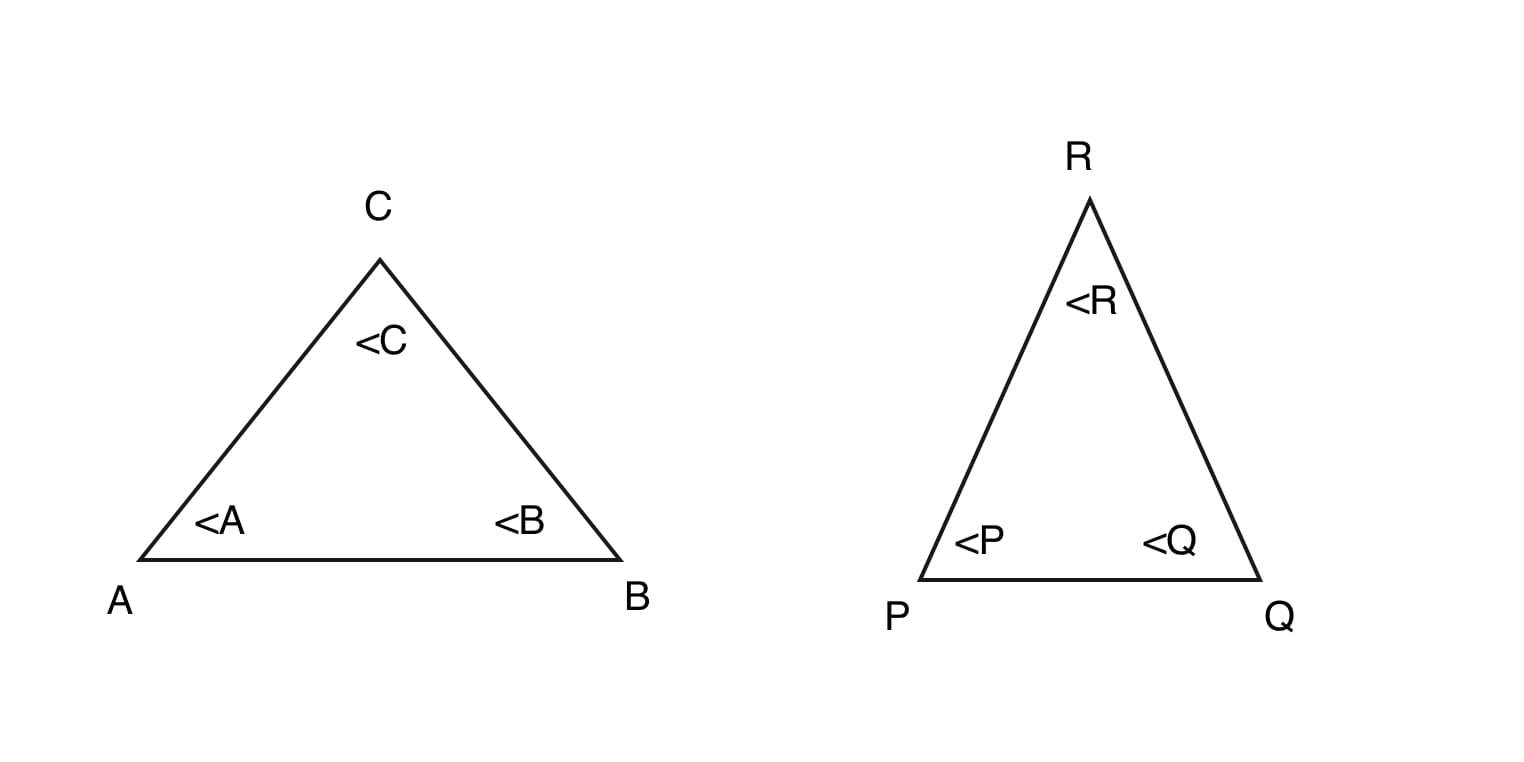Related Articles
Program to check similarity of given two triangles
• Last Updated : 28 Dec, 2018

Given four array of 3 numbers each which represents sides and angles of two triangles. The task is to check if two triangles are similar or not. If it is similar, print the theorem by which it is.

Examples:

```Input : side1 = [2, 3, 3] angle1 = [80, 60, 40]
side2 = [4, 6, 6]  angle2 = [40, 60, 80]
Output: Triangles are similar by SSS AAA SAS

Input : side1 = [2, 3, 4] angle1 = [85, 45, 50]
side2 = [4, 6, 6]  angle2 = [40, 60, 80]
Output: Triangles are not similar
```

## Recommended: Please try your approach on {IDE} first, before moving on to the solution.

Similar triangles are two or more triangles that have all corresponding angles that are equal and all corresponding sides that are proportionate. It does not matter what direction the triangles are facing. Their size does not matter as long as each side is proportionate. The similarity of triangles can be proved by the following theorems:

1. Side-Side-Side (SSS) similarity criteria :
If all the sides of a triangle are proportional to the corresponding sides of another triangle then the triangles are said to be similar by the property of Side-Side-Side (SSS).
In a triangle ABC and PQR if, AB/PQ = BC/QR = CA/RP triangles are similar.2. Side-Angle-Side (SAS) similarity criteria :
If two sides of the two triangles are proportional and the angle between them is same in both triangle then the triangles are said to be similar by the property of Side-Angle-Side (SAS).
In a triangle ABC and PQR if, AB/PQ = BC/QR and=triangles are similar.3. Angle-Angle-Angle (AAA) similarity criteria :
If all the angles of a triangle are equal to the corresponding angles of another triangle then the triangles are said to be similar by the property of Angle-Angle-Angle (AAA).
In a triangle ABC and PQR if=,=and=then triangles are similar.Below is the implementation of the above approach:

## C++

 `// C++ program to check  ` `// similarity between  ` `// two triangles. ` `#include ` `using` `namespace` `std; ` ` `  `//Function for AAA similarity ` `int` `simi_aaa(``int` `a1[], ``int` `a2[]) ` `{ ` `    ``sort(a1, a1 + 3); ` `    ``sort(a2, a2 + 3); ` `     `  `    ``// Check for AAA ` `    ``if` `(a1 == a2 &&  ` `        ``a1 == a2 &&  ` `        ``a1 == a2) ` `    ``return` `1; ` `    ``else` `    ``return` `0; ` `     `  `} ` ` `  `// Function for ` `// SAS similarity ` `int` `simi_sas(``int` `s1[], ``int` `s2[],  ` `             ``int` `a1[], ``int` `a2[]) ` `{ ` `    ``sort(a1, a1 + 3); ` `    ``sort(a2, a2 + 3); ` `    ``sort(s1, s1 + 3); ` `    ``sort(s2, s2 + 3); ` `     `  `    ``// Check for SAS ` `     `  `    ``// angle b / w two smallest ` `    ``// sides is largest. ` `    ``if``( s1 / s2 == s1 /  ` `                         ``s2) ` `    ``{ ` `        ``// since we take angle ` `        ``// b / w the sides. ` `        ``if` `(a1 == a2)          ` `            ``return` `1; ` `    ``} ` `    ``if` `(s1 / s2 == s1 / ` `                         ``s2) ` `    ``{ ` `        ``if` `(a1 == a2) ` `            ``return` `1; ` `        ``}  ` `    ``if` `(s1 / s2 == s1 /     ` `                         ``s2) ` `    ``{ ` `        ``if``(a1 == a2) ` `            ``return` `1; ` `        ``} ` `    ``return` `0; ` `} ` ` `  `// Function for SSS similarity ` `int` `simi_sss(``int` `s1[], ``int` `s2[]) ` `{ ` `    ``sort(s1, s1 + 3); ` `    ``sort(s2, s2 + 3); ` `     `  `    ``// Check for SSS ` `    ``if``(s1 / s2 == s1 / s2 &&  ` `       ``s1 / s2 == s1 / s2 &&  ` `       ``s1 / s2 == s1 / s2) ` `        ``return` `1; ` `     `  `    ``return` `0; ` `} ` ` `  `// Driver Code ` `int` `main() ` `{ ` `    ``int` `s1[] = {2, 3, 3}; ` `    ``int` `s2[] = {4, 6, 6}; ` `         `  `    ``int` `a1[] = {80, 60, 40}; ` `    ``int` `a2[] = {40, 60, 80}; ` ` `  `    ``// function call for ` `    ``// AAA similarity ` `    ``int` `aaa = simi_aaa(a1, a2);  ` ` `  `    ``// function call for ` `    ``// SSS similarity  ` `    ``int` `sss = simi_sss(s1, s2) ; ` ` `  `    ``// function call for ` `    ``// SAS similarity  ` `    ``int` `sas = simi_sas(s1, s2,  ` `                       ``a1, a2) ; ` ` `  `    ``// Check if triangles ` `    ``// are similar or not  ` `    ``if``(aaa == 1 ||  ` `       ``sss == 1 || sas == 1) ` `    ``{ ` `        ``cout << ``"Triangles are "`  `             ``<< ``"similar by "``; ` `        ``if``(aaa == 1) cout << ``"AAA "``; ` `        ``if``(sss == 1) cout << ``"SSS "``; ` `        ``if``(sas == 1) cout << ``"SAS."``; ` `    ``} ` `     `  `    ``else` `        ``cout << ``"Triangles are "`  `             ``<< ``"not similar"``; ` `    ``return` `0; ` `} ` ` `  `// This code is contributed ` `// by Arnab Kundu `

## Java

 `// Java program to check  ` `// similarity between  ` `// two triangles. ` `import` `java.util.*; ` `class` `GFG1 ` `{ ` `     `  `// Function for ` `// AAA similarity ` `static` `int` `simi_aaa(``int` `a1[],  ` `                    ``int` `a2[]) ` `{ ` `    ``Arrays.sort(a1); ` `    ``Arrays.sort(a2); ` `     `  `    ``// Check for AAA ` `    ``if` `(a1[``0``] == a2[``0``] &&  ` `        ``a1[``1``] == a2[``1``] && ` `        ``a1[``2``] == a2[``2``]) ` `    ``return` `1``; ` `    ``else` `    ``return` `0``; ` `     `  `} ` ` `  `// Function for ` `// SAS similarity ` `static` `int` `simi_sas(``int` `s1[], ``int` `s2[],  ` `                    ``int` `a1[], ``int` `a2[]) ` `{ ` `    ``Arrays.sort(a1); ` `    ``Arrays.sort(a2); ` `    ``Arrays.sort(s1); ` `    ``Arrays.sort(s2); ` `     `  `    ``// Check for SAS ` `     `  `    ``// angle b / w two smallest ` `    ``// sides is largest. ` `    ``if``(s1[``0``] / s2[``0``] == s1[``1``] / s2[``1``]) ` `    ``{ ` `        ``// since we take angle ` `        ``// b / w the sides. ` `        ``if` `(a1[``2``] == a2[``2``])          ` `            ``return` `1``; ` `    ``} ` `    ``if` `(s1[``1``] / s2[``1``] == s1[``2``] / s2[``2``]) ` `    ``{ ` `        ``if` `(a1[``0``] == a2[``0``]) ` `            ``return` `1``; ` `    ``}  ` `    ``if` `(s1[``2``] / s2[``2``] == s1[``0``] / s2[``0``]) ` `    ``{ ` `        ``if``(a1[``1``] == a2[``1``]) ` `            ``return` `1``; ` `    ``} ` `    ``return` `0``; ` `} ` ` `  `// Function for  ` `// SSS similarity ` `static` `int` `simi_sss(``int` `s1[], ` `                    ``int` `s2[]) ` `{ ` `    ``Arrays.sort(s1); ` `    ``Arrays.sort(s2); ` `     `  `    ``// Check for SSS ` `    ``if``(s1[``0``] / s2[``0``] == s1[``1``] / s2[``1``] &&  ` `       ``s1[``1``] / s2[``1``] == s1[``2``] / s2[``2``] &&  ` `       ``s1[``2``] / s2[``2``] == s1[``0``] / s2[``0``]) ` `        ``return` `1``; ` `     `  `    ``return` `0``; ` `} ` ` `  `// Driver Code ` `public` `static` `void` `main(String args[]) ` `{ ` `    ``int` `s1[] = {``2``, ``3``, ``3``}; ` `    ``int` `s2[] = {``4``, ``6``, ``6``}; ` `         `  `    ``int` `a1[] = {``80``, ``60``, ``40``}; ` `    ``int` `a2[] = {``40``, ``60``, ``80``}; ` ` `  `    ``// function call for ` `    ``// AAA similarity ` `    ``int` `aaa = simi_aaa(a1, a2);  ` ` `  `    ``// function call for ` `    ``// SSS similarity  ` `    ``int` `sss = simi_sss(s1, s2) ; ` ` `  `    ``// function call for  ` `    ``// SAS similarity  ` `    ``int` `sas = simi_sas(s1, s2,  ` `                       ``a1, a2) ; ` ` `  `    ``// Check if triangles  ` `    ``// are similar or not  ` `    ``if``(aaa == ``1` `||  ` `       ``sss == ``1` `|| sas == ``1``) ` `    ``{ ` `        ``System.out.print(``"Triangles are "` `+  ` `                         ``"similar by "``); ` `        ``if``(aaa == ``1``) System.out.print(``"AAA "``); ` `        ``if``(sss == ``1``) System.out.print(``"SSS "``); ` `        ``if``(sas == ``1``) System.out.print(``"SAS."``); ` `    ``} ` `    ``else` `        ``System.out.println(``"Triangles are "` `+  ` `                           ``"not similar"``); ` `} ` `} ` ` `  `// This code is contributed  ` `// by Arnab Kundu `

## Python

 `# Python program to check  ` `# similarity between two triangles. ` ` `  `# Function for AAA similarity ` `def` `simi_aaa(a1, a2):              ` `    ``a1 ``=` `[``float``(i) ``for` `i ``in` `a1] ` `    ``a2 ``=` `[``float``(i) ``for` `i ``in` `a2] ` `    ``a1.sort() ` `    ``a2.sort() ` `     `  `    ``# Check for AAA ` `    ``if` `a1[``0``] ``=``=` `a2[``0``] ``and` `a1[``1``] ``=``=` `a2[``1``] ``and` `a1[``2``] ``=``=` `a2[``2``]: ` `        ``return` `1` `    ``return` `0` ` `  `# Function for SAS similarity ` `def` `simi_sas(s1, s2, a1, a2):  ` `     `  `    ``s1 ``=` `[``float``(i) ``for` `i ``in` `s1] ` `    ``s2 ``=` `[``float``(i) ``for` `i ``in` `s2] ` `    ``a1 ``=` `[``float``(i) ``for` `i ``in` `a1] ` `    ``a2 ``=` `[``float``(i) ``for` `i ``in` `a2] ` `     `  `    ``s1.sort() ` `    ``s2.sort() ` `    ``a1.sort() ` `    ``a2.sort() ` `     `  `    ``# Check for SAS ` `     `  `    ``# angle b / w two smallest sides is largest. ` `    ``if` `s1[``0``] ``/` `s2[``0``] ``=``=` `s1[``1``] ``/` `s2[``1``]: ` `         `  `        ``# since we take angle b / w the sides. ` `        ``if` `a1[``2``] ``=``=` `a2[``2``]:          ` `            ``return` `1` `             `  `    ``if` `s1[``1``] ``/` `s2[``1``] ``=``=` `s1[``2``] ``/` `s2[``2``]: ` `        ``if` `a1[``0``] ``=``=` `a2[``0``]: ` `            ``return` `1` `             `  `    ``if` `s1[``2``] ``/` `s2[``2``] ``=``=` `s1[``0``] ``/` `s2[``0``]: ` `        ``if` `a1[``1``] ``=``=` `a2[``1``]: ` `            ``return` `1` `     `  `    ``return` `0` ` `  `# Function for SSS similarity ` `def` `simi_sss(s1, s2):  ` `     `  `    ``s1 ``=` `[``float``(i) ``for` `i ``in` `s1] ` `    ``s2 ``=` `[``float``(i) ``for` `i ``in` `s2]  ` `    ``s1.sort() ` `    ``s2.sort()  ` `     `  `    ``# Check for SSS ` `    ``if``(s1[``0``] ``/` `s2[``0``] ``=``=` `s1[``1``] ``/` `s2[``1``]  ` `        ``and` `s1[``1``] ``/` `s2[``1``] ``=``=` `s1[``2``] ``/` `s2[``2``]  ` `        ``and` `s1[``2``] ``/` `s2[``2``] ``=``=` `s1[``0``] ``/` `s2[``0``]): ` `        ``return` `1` `     `  `    ``return` `0` `     `  ` `  `# Driver Code  ` `s1 ``=` `[``2``, ``3``, ``3``] ` `s2 ``=` `[``4``, ``6``, ``6``] ` `         `  `a1 ``=` `[``80``, ``60``, ``40``] ` `a2 ``=` `[``40``, ``60``, ``80``] ` ` `  `# function call for AAA similarity ` `aaa ``=` `simi_aaa(a1, a2)  ` ` `  `# function call for SSS similarity  ` `sss ``=` `simi_sss(s1, s2)  ` ` `  `# function call for SAS similarity  ` `sas ``=` `simi_sas(s1, s2, a1, a2)  ` ` `  `# Check if triangles are similar or not  ` `if` `aaa ``or` `sss ``or` `sas:  ` `    ``print` `"Triangles are similar by"``, ` `    ``if` `aaa: ``print` `"AAA"``,  ` `    ``if` `sss: ``print` `"SSS"``,  ` `    ``if` `sas: ``print` `"SAS"` `else``: ``print` `"Triangles are not similar"` `            `

## C#

 `// C# program to check  ` `// similarity between  ` `// two triangles.  ` ` `  `using` `System; ` ` `  `class` `GFG1  ` `{  ` `     `  `// Function for  ` `// AAA similarity  ` `static` `int` `simi_aaa(``int` `[] a1,  ` `                    ``int` `[] a2)  ` `{  ` `    ``Array.Sort(a1);  ` `    ``Array.Sort(a2);  ` `     `  `    ``// Check for AAA  ` `    ``if` `(a1 == a2 &&  ` `        ``a1 == a2 &&  ` `        ``a1 == a2)  ` `    ``return` `1;  ` `    ``else` `    ``return` `0;  ` `     `  `}  ` ` `  `// Function for  ` `// SAS similarity  ` `static` `int` `simi_sas(``int` `[] s1, ``int` `[] s2,  ` `                    ``int` `[] a1, ``int` `[] a2)  ` `{  ` `    ``Array.Sort(a1);  ` `    ``Array.Sort(a2);  ` `    ``Array.Sort(s1);  ` `    ``Array.Sort(s2);  ` `     `  `    ``// Check for SAS  ` `     `  `    ``// angle b / w two smallest  ` `    ``// sides is largest.  ` `    ``if``(s1 / s2 == s1 / s2)  ` `    ``{  ` `        ``// since we take angle  ` `        ``// b / w the sides.  ` `        ``if` `(a1 == a2)          ` `            ``return` `1;  ` `    ``}  ` `    ``if` `(s1 / s2 == s1 / s2)  ` `    ``{  ` `        ``if` `(a1 == a2)  ` `            ``return` `1;  ` `    ``}  ` `    ``if` `(s1 / s2 == s1 / s2)  ` `    ``{  ` `        ``if``(a1 == a2)  ` `            ``return` `1;  ` `    ``}  ` `    ``return` `0;  ` `}  ` ` `  `// Function for  ` `// SSS similarity  ` `static` `int` `simi_sss(``int` `[] s1,  ` `                    ``int` `[] s2)  ` `{  ` `    ``Array.Sort(s1);  ` `    ``Array.Sort(s2);  ` `     `  `    ``// Check for SSS  ` `    ``if``(s1 / s2 == s1 / s2 &&  ` `    ``s1 / s2 == s1 / s2 &&  ` `    ``s1 / s2 == s1 / s2)  ` `        ``return` `1;  ` `     `  `    ``return` `0;  ` `}  ` ` `  `// Driver Code  ` `public` `static` `void` `Main()  ` `{  ` `    ``int` `[] s1 = {2, 3, 3};  ` `    ``int` `[] s2 = {4, 6, 6};  ` `         `  `    ``int` `[] a1 = {80, 60, 40};  ` `    ``int` `[] a2 = {40, 60, 80};  ` ` `  `    ``// function call for  ` `    ``// AAA similarity  ` `    ``int` `aaa = simi_aaa(a1, a2);  ` ` `  `    ``// function call for  ` `    ``// SSS similarity  ` `    ``int` `sss = simi_sss(s1, s2) ;  ` ` `  `    ``// function call for  ` `    ``// SAS similarity  ` `    ``int` `sas = simi_sas(s1, s2,  ` `                    ``a1, a2) ;  ` ` `  `    ``// Check if triangles  ` `    ``// are similar or not  ` `    ``if``(aaa == 1 ||  ` `    ``sss == 1 || sas == 1)  ` `    ``{  ` `        ``Console.Write(``"Triangles are "` `+  ` `                        ``"similar by "``);  ` `        ``if``(aaa == 1) Console.Write(``"AAA "``);  ` `        ``if``(sss == 1) Console.Write(``"SSS "``);  ` `        ``if``(sas == 1) Console.Write(``"SAS."``);  ` `    ``}  ` `    ``else` `        ``Console.WriteLine(``"Triangles are "` `+  ` `                        ``"not similar"``);  ` `}  ` `}  ` ` `  `// This code is contributed  ` `// by Ryuga `

## PHP

 ` `

Output:

```Triangles are similar by AAA SSS SAS
```

Attention reader! Don’t stop learning now. Get hold of all the important DSA concepts with the DSA Self Paced Course at a student-friendly price and become industry ready.

My Personal Notes arrow_drop_up
Recommended Articles
Page :